Homework Help Question & Answers

# 4. (a) Define when two elements of a group are conjugate to each other. State and de- duce the cl...

Please show all steps clearly.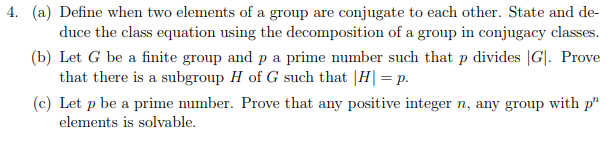4. (a) Define when two elements of a group are conjugate to each other. State and de- duce the class equation using the decomposition of a group in conjugacy classes (b) Let G be a finite group and p a prime number such that p divides G. Prove that there is a subgroup H of G such that |H p. (c) Let p be a prime number. Prove that any positive integer n, any group with p" elements is solvable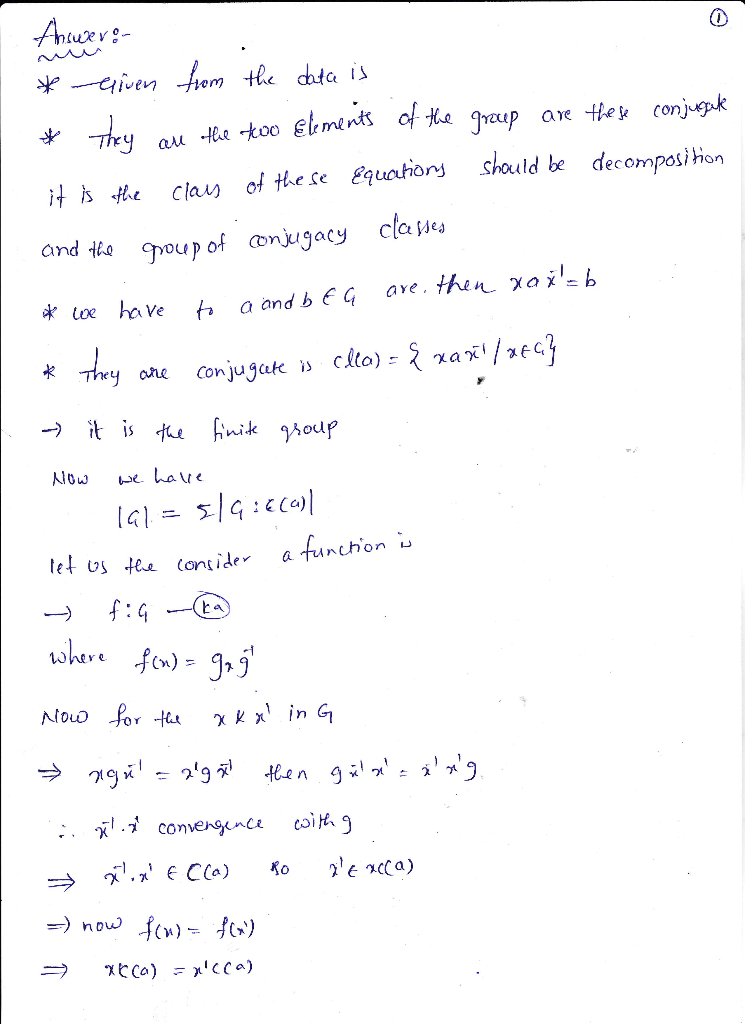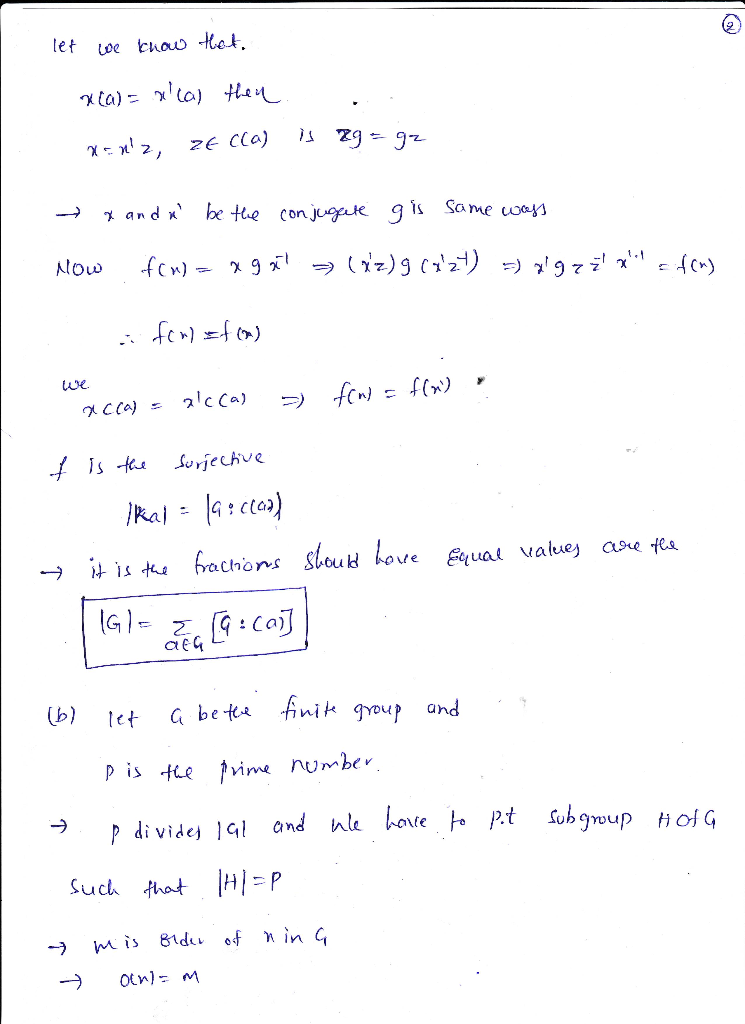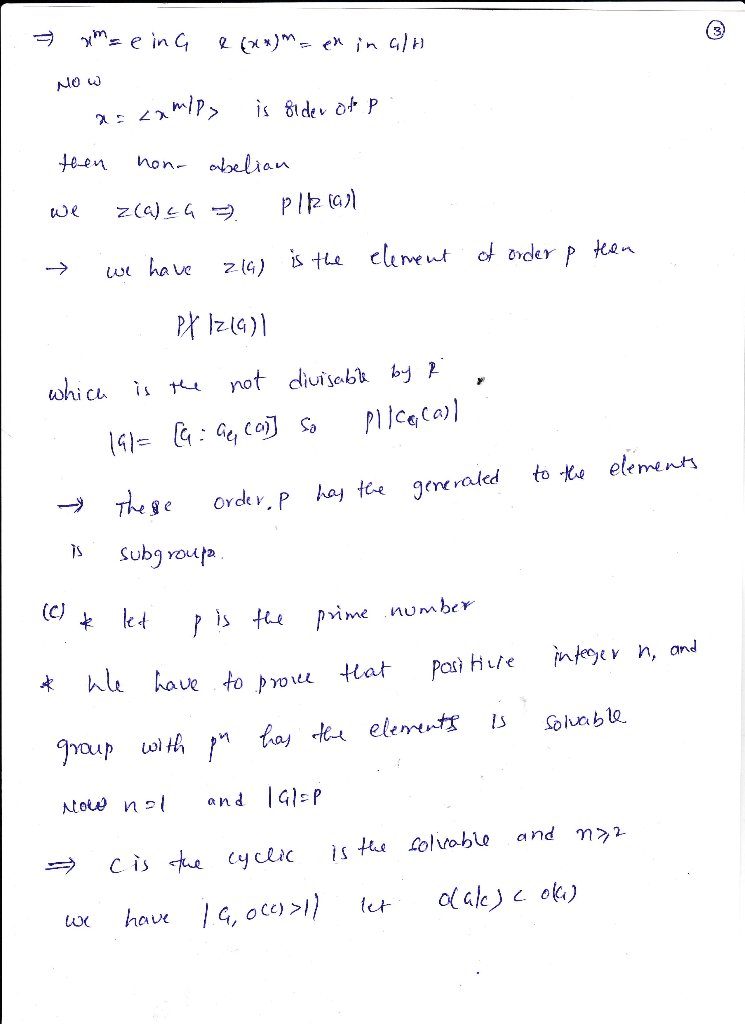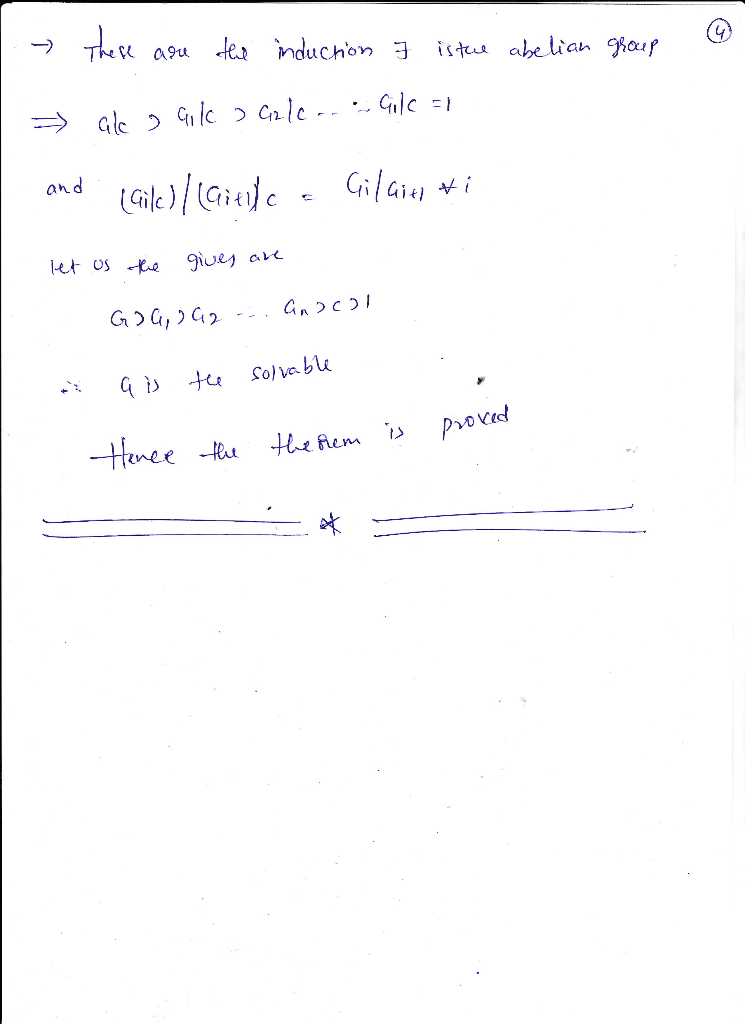##### Add Answer of: 4. (a) Define when two elements of a group are conjugate to each other. State and de- duce the cl...
More Homework Help Questions Additional questions in this topic.

• #### 1) The albatross as well as the spirits, the angels and the sea monsters are other supernatural elements (Is it correct or is it better to say "Other supernatural elements include

Need Online Homework Help?

Get FREE EXPERT Answers
WITHIN MINUTES
Related Questions